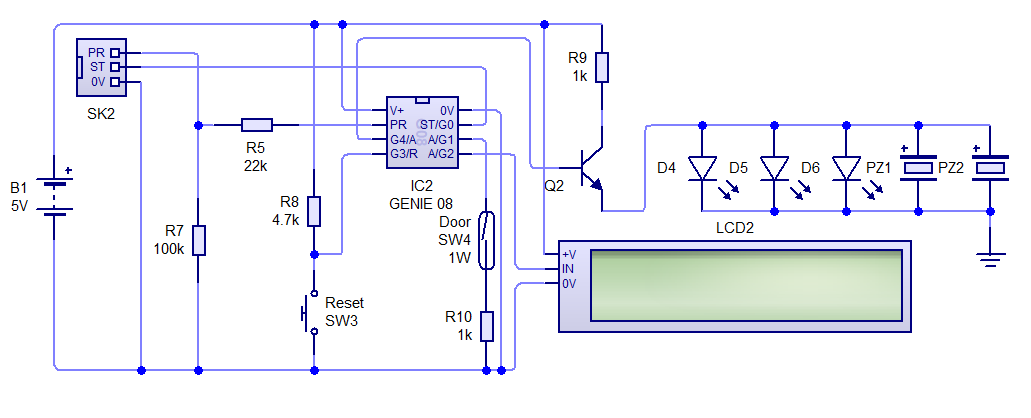# Is there a component that doesn't let anything below a certain voltage through?

I was wondering if there was a component that would only let current flow if it was above a predetermined voltage.

I am building a subsystem for my project, which is an open door sensor that uses a microcontroller. I am running the circuit at 5 V, and I want an LCD display to display when it is time to change the batteries. The circuit will not have an external power supply to reference the voltage to when testing the batteries.This is my circuit so far and it only needs this last bit. Whilst the microcontroller can run at as low as 3 V, the LCD needs 4.5 V - 5.5 V to work, so 4.5 V will be my minimum voltage, but I would like to know in advance, so maybe a bit higher.

Any help in understanding this would be greatly appreciated.

• Anyway, you're probably still barking up the wrong tree – describe exactly what problem your "battery tester subsystem" is supposed to solve – arbitrary bets this is not the solution. Apr 21, 2020 at 21:40
• Anything from a zener diode to a comparator could answer your question. We need more details about your circuit and exactly what you are trying to do to give a helpful answer. Apr 21, 2020 at 22:36
• There are a number of existing questions here which deal with this topic. 1, 2, 3, etc. The basic idea typically is to divide your battery voltage down so that it's in the range of your microcontroller's ADC input. Apr 21, 2020 at 23:40
• @MaltedWheaties most microcontrollers with an ADC will also have an internal voltage reference to solve this problem. Apr 23, 2020 at 7:51

You'll want to use a voltage reference along with an ADC or analog comparator. Voltage references work kind of like an LDO voltage regulator; the difference is that they put out a very stable and accurate voltage (but very little current). The following circuit will do the job:The two resistors will supply half the battery voltage to the comparator. The chosen voltage reference is 1.6 V (half of a LiPo's very low battery level of 3.2 V ). The comparator will output true if Vbat1 drops below 3.2 V. Alternatively, one could use an ADC that allows a reference voltage as an input:In this case the voltage reference is chosen to be over half the maximum battery voltage (2.5 V × 2 = 5.0 V). The output of the voltage divider is measured by the ADC using 2.5 V as the top of the measurement range. To get the actual voltage plug the ADC output value $$\N\$$ into the following: $$V_{bat} = { { 2 \times N \times V_{ref} } \over { 2^{resolution} - 1 } }$$ The result is the measured battery voltage $$\V_{bat}\$$ in volts. $$\V_{ref}\$$ is the value of the voltage reference (in volts) and $$\resolution\$$ is the resolution of your ADC (in bits). Many popular microcontrollers and development boards have built-in ADCs with support for external voltage references (the Teensy 3.x and Feather M0 boards come to mind).

For either of the above you should choose a voltage reference that's appropriate for your power source. LiPo batteries, for example, typically max out at 4.2 V, spend most of their useful life at 3.7 V, and cut out completely at 3.0 V. Alkaline batteries, on the other hand, start out at 1.5 V then end their useful life around 1.15 V (IIRC). Your typical NiMH will start out at 1.3 V and dip to a tad under 1 V before they're unusable (again, IIRC).

I used LiPo batteries for the examples above; regardless of the battery technology you use you'll want to feed it to a 5 V regulator2 that can then power the rest of your circuit.

1 $$\V_{bat}\$$ is the raw battery voltage, not the output of your voltage regulator.

2 For LiPo batteries you'd use a boost regulator.

4.5V will be my minimum voltage, but I would like to know in advance, so maybe a bit higher.

You could use a Zener diode and resistor. The Zener diode drops a relatively fixed voltage, so as the power supply voltage drops the zener output voltage drops proportionally faster. The MCU has an ADC which measures the ratio between its input and the power supply voltage, so the ADC count reduces as the battery voltage drops.simulate this circuit – Schematic created using CircuitLab

I simulated this circuit in LTspice with battery voltage dropping from 6 V to 4 V, and got the following results (blue = battery volts, green = ADC input, red = ADC count):-8 bit ADC count varied from 71 at 6V to 20 at 4 V, an average of ~1 count per 40 mV. At 4.8 V it was 41. This should be accurate enough to show battery voltage with a resolution of 0.1 V, or as a bar display with 10 or more segments.

Only one problem - you appear to be already using all the pins on your MCU! You may be able to reconfigure the PR pin for ADC input. If so then you will need a jumper or switch to select between programming the MCU and reading the battery voltage.